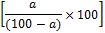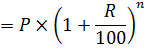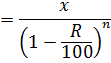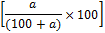New Students Offer - Use Code HELLO

# 10 Important Percentage Tricks With Examples

Percentage means per cent or per 100
A percentage is a number or ratio which is expressed as a fraction of 100.
Ex. 30%=30/100

#### 1.

If we have to convert a fraction into % than that number is multiply by 100.
Example
Change 2/5 into percentage.
=2/5×100
=40%

#### 2.

If we have to convert % into fraction than that number is multipy by 100
Example
Chnage 25% into fraction.
=25/100
=1/4

#### 3.

A is% of 'B'.
Example
If 'X' is a% more than 'Y', then 'Y' is% less than 'X'.

Example

If the salary of Ram is 25% more than shyam's salary. Find how much % Shyam's salary is less than Ram's salary?=20%

#### 5.

If 'X' is a% less than 'Y', then 'Y' is% more than 'X'.

Example
If the population is 'P' and it is incearsed by 'R%' every year then the population of village after 'n' years isExample
If the population is 'P' and it is incearsed by 'R%' every year then the population before 'n' years isExample
If the price of a machine is x rs. and it is depriciated by 'R%' every year then the price of machine after 'n' years isExample
If the price of a machine is x rs. and it is depriciated by 'R%' every year then the price of machine before 'n' years isExample
If the price of any foodstuff is increased by 'a%' then the comsumption of it will be decreased by% so that the expenses of this item is remain same.

Example